# Analysis for the Dog Survey

The main task is to get unbiassed estimates of total dog population, with confidence intervals. The secondary task is to get additional information about dog health status, etc.
As the square blocks were sampled with different probabilities in each level, different sample weights need to be applied.

We use the R package `survey` for the analysis.

First we read in the two separate datasets, `blocks-for-data.csv` which describes the blocks (latitude, longitude, time of day, team number etc.) and the longer database `data.csv` which lists every single dog, keyed by the block id and level id. Note that the block identifier in both datasets is the combination of both the number and the level, as the numbers repeat inside each level.
Then we merge the databases.

We have to make sure we treat the no-dogs case correctly, i.e. rows in data where one person found no dogs in a square. We dont code nepoznato in this case; everything else is missing.

``````setwd("~/Dropbox/research/DogsTrust/data")
library(survey)  #downloads tiles from osm
library(ggplot2)  #
library(xtable)  #
library(OpenStreetMap)  #
# The main statistic of interest is the number of dogs per square. So we
# aggregate the data to get this.

opts_chunk\$set(fig.width = 12)

data = read.csv("data.csv", header = T, quote = "'")
data\$counter = ifelse(data\$dogNumber != 0, 1, 0)  #just one for every dog, in order to calculate means and totals
## make sure we treat the no-dogs case correctly, i.e. rows in data where
## one person found no dogs in a square.  in this case, data entry for
## dognumber is 0 not e.g. nepoznato or missing; all other variables apart
## from team member entered as missing.

datag = aggregate(data\$counter, by = list(level = data\$level, square = data\$square),
FUN = sum, T)  #
blocks = read.csv("blocks-for-data.csv", header = T)
data = data[!(data\$level == 2 & data\$square %in% c(2, 3, 4)), ]  #randomly deleting two out of the six blocks in l2
comb = merge(data, blocks)
# combg=merge(datag,blocks)
l0sampleTol0total = 0.024  #l0samplearea/l0totalarea
l1sampleTol1total = 0.398  #l1samplearea/l1totalarea
l2sampleTol2total = 1.682 * 0.5  #but fpc cannot go over 1!! So the level2 scores are not weighted down quite as much as they should be. However as the mean score does not significantly differ between levels (!) - see below - we can ignore this.

comb\$fpc = sapply(comb\$level, function(x) switch(x + 1, l0sampleTol0total,
l1sampleTol1total, l2sampleTol2total))
combg = aggregate(comb[, c("counter", "fpc")], list(square = comb\$square,
level = comb\$level, group = comb\$group), function(x) sum(x, na.rm = T))  #in case we need the data aggregated by block
combg\$fpc = sapply(combg\$level, function(x) switch(x + 1, l0sampleTol0total,
l1sampleTol1total, l2sampleTol2total))
# so the variable called counter in this aggregated data set show the
# total number of dogs per square

ggplot(combg, aes(counter)) + geom_density()
``````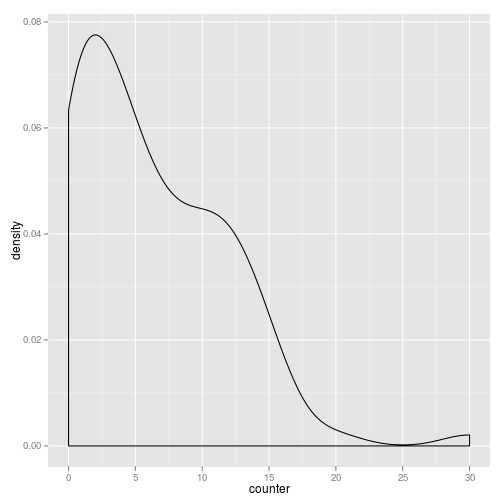``````ggplot(combg, aes(counter, group = group)) + geom_density(aes(colour = group))
``````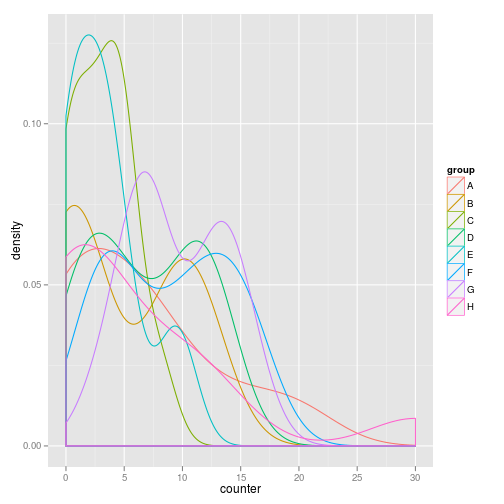``````# In levels 1 and 2, there seems to be a tendency to find either less than
# 5 dogs or between 10 and 15 dogs. Very interesting - pack size?
ggplot(combg, aes(counter)) + geom_density(aes(colour = factor(level)))
``````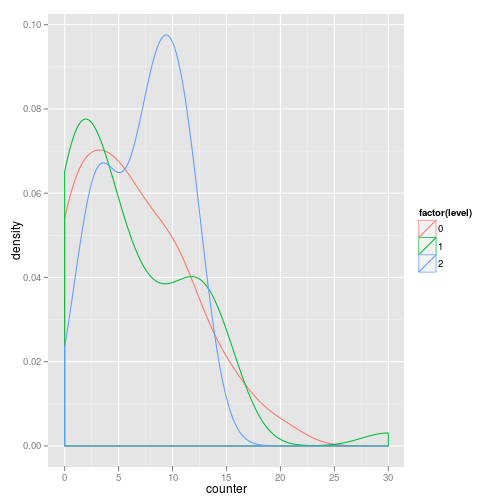``````# very interesting - although the mean number of dogs does not differ
# significantly between levels, in fact there are fewer squares with no
# dogs in level2. This is counterbalanced by the fact that there are
# occasionally even more dogs in the other levels.

combgc = aggregate(comb[, c("counter", "fpc")], list(square = comb\$square,
level = comb\$level, clan = comb\$clan, group = comb\$group, day = comb\$day,
hour = comb\$hour), function(x) sum(x, na.rm = T))  #in case we need the data aggregated by block/volunteer
combgc\$fpc = sapply(combgc\$level, function(x) switch(x + 1, l0sampleTol0total,
l1sampleTol1total, l2sampleTol2total))
# so the variable called counter in this aggregated data set shows the
# total number of dogs per square and per volunteer

combl = aggregate(combg[, c("counter", "fpc")], list(level = combg\$level),
function(x) mean(x, na.rm = T))  #in case we need the data aggregated by level
combl\$fpc = sapply(combl\$level, function(x) switch(x + 1, l0sampleTol0total,
l1sampleTol1total, l2sampleTol2total))
combl  #average number of dogs per block in each level - slightly more in level 2 as in level 1, which is slightly more than level 0
``````
``````##   level counter   fpc
## 1     0   6.359 0.024
## 2     1   6.075 0.398
## 3     2   7.333 0.841
``````

## Basic results

How does the number of dogs counted vary by day, hour, group etc?

``````
ggplot(data = comb, aes(paste(as.character(day), as.character(hour)),
dogNumber)) + stat_summary(fun.y = sum, size = 6, aes(colour = factor(hour)),
geom = "point") + coord_flip() + scale_colour_brewer(type = "qual") + ylim(c(0,
70))  #doesnt look like day or hour influence the count much.
``````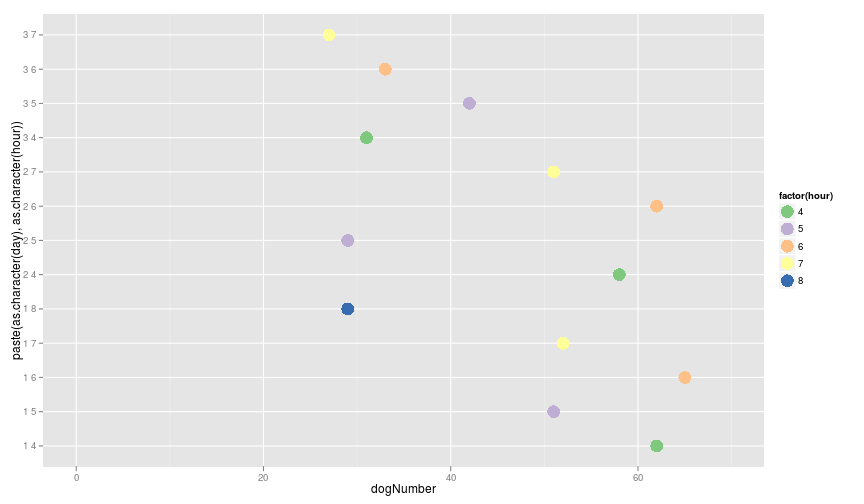``````ggplot(data = comb, aes(paste(group, clan), dogNumber)) + stat_summary(fun.y = sum,
size = 6, aes(colour = group), geom = "point") + coord_flip() + scale_colour_brewer(type = "qual")  #this shows that the number of dogs found varies widely between member and group. It doesn't look like there were groups which all found a lot of dogs.
``````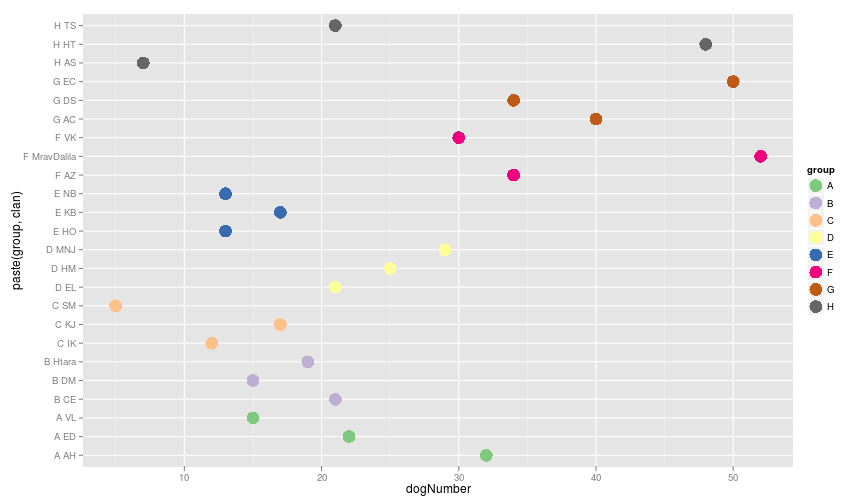``````#
lm = glm(data = combgc, counter ~ level)
summary(lm)  #level is not important
``````
``````##
## Call:
## glm(formula = counter ~ level, data = combgc)
##
## Deviance Residuals:
##    Min      1Q  Median      3Q     Max
## -2.198  -2.156  -1.156   0.844  20.823
##
## Coefficients:
##             Estimate Std. Error t value Pr(>|t|)
## (Intercept)    2.156      0.248    8.70  3.2e-16 ***
## level          0.021      0.302    0.07     0.94
## ---
## Signif. codes:  0 '***' 0.001 '**' 0.01 '*' 0.05 '.' 0.1 ' ' 1
##
## (Dispersion parameter for gaussian family taken to be 7.337)
##
##     Null deviance: 1988.2  on 272  degrees of freedom
## Residual deviance: 1988.2  on 271  degrees of freedom
## AIC: 1323
##
## Number of Fisher Scoring iterations: 2
##
``````
``````lm0 = glm(data = combgc, counter ~ level + factor(day) * factor(hour))
summary(lm0)
``````
``````##
## Call:
## glm(formula = counter ~ level + factor(day) * factor(hour), data = combgc)
##
## Deviance Residuals:
##    Min      1Q  Median      3Q     Max
## -2.968  -1.604  -0.549   1.207  20.032
##
## Coefficients: (2 not defined because of singularities)
##                            Estimate Std. Error t value Pr(>|t|)
## (Intercept)                 2.54895    0.58718    4.34    2e-05 ***
## level                       0.05501    0.31394    0.18     0.86
## factor(day)2                0.18938    0.81248    0.23     0.82
## factor(day)3               -1.17987    0.80090   -1.47     0.14
## factor(hour)5              -0.45146    0.78365   -0.58     0.57
## factor(hour)6               0.11812    0.78365    0.15     0.88
## factor(hour)7              -0.11205    0.81062   -0.14     0.89
## factor(hour)8              -0.17354    0.95937   -0.18     0.86
## factor(day)2:factor(hour)5 -0.78661    1.16290   -0.68     0.50
## factor(day)3:factor(hour)5  0.87498    1.12530    0.78     0.44
## factor(day)2:factor(hour)6  0.05664    1.14681    0.05     0.96
## factor(day)3:factor(hour)6  0.03707    1.13874    0.03     0.97
## factor(day)2:factor(hour)7 -0.09278    1.17412   -0.08     0.94
## factor(day)3:factor(hour)7 -0.00275    1.16035    0.00     1.00
## factor(day)2:factor(hour)8       NA         NA      NA       NA
## factor(day)3:factor(hour)8       NA         NA      NA       NA
## ---
## Signif. codes:  0 '***' 0.001 '**' 0.01 '*' 0.05 '.' 0.1 ' ' 1
##
## (Dispersion parameter for gaussian family taken to be 7.351)
##
##     Null deviance: 1988.2  on 272  degrees of freedom
## Residual deviance: 1903.8  on 259  degrees of freedom
## AIC: 1335
##
## Number of Fisher Scoring iterations: 2
##
``````
``````lm1 = glm(data = combgc, counter ~ level + group + factor(day) *
factor(hour))
summary(lm1)
``````
``````##
## Call:
## glm(formula = counter ~ level + group + factor(day) * factor(hour),
##     data = combgc)
##
## Deviance Residuals:
##    Min      1Q  Median      3Q     Max
## -3.649  -1.521  -0.545   1.041  20.152
##
## Coefficients: (2 not defined because of singularities)
##                            Estimate Std. Error t value Pr(>|t|)
## (Intercept)                 2.59330    0.70578    3.67  0.00029 ***
## level                       0.08225    0.32240    0.26  0.79885
## groupB                     -0.40972    0.67039   -0.61  0.54164
## groupC                     -1.04429    0.69018   -1.51  0.13152
## groupD                      0.00507    0.67849    0.01  0.99404
## groupE                     -1.04882    0.64214   -1.63  0.10365
## groupF                      0.91027    0.64754    1.41  0.16103
## groupG                      1.14489    0.64282    1.78  0.07611 .
## groupH                     -0.04840    0.65221   -0.07  0.94090
## factor(day)2                0.14496    0.79247    0.18  0.85500
## factor(day)3               -1.17271    0.77828   -1.51  0.13311
## factor(hour)5              -0.44805    0.76132   -0.59  0.55671
## factor(hour)6               0.11472    0.76132    0.15  0.88035
## factor(hour)7              -0.10572    0.78965   -0.13  0.89360
## factor(hour)8              -0.47781    0.94708   -0.50  0.61435
## factor(day)2:factor(hour)5 -0.73182    1.13554   -0.64  0.51986
## factor(day)3:factor(hour)5  0.91730    1.09324    0.84  0.40223
## factor(day)2:factor(hour)6 -0.03839    1.11920   -0.03  0.97266
## factor(day)3:factor(hour)6  0.02938    1.10713    0.03  0.97885
## factor(day)2:factor(hour)7 -0.09305    1.14580   -0.08  0.93534
## factor(day)3:factor(hour)7 -0.09477    1.12994   -0.08  0.93323
## factor(day)2:factor(hour)8       NA         NA      NA       NA
## factor(day)3:factor(hour)8       NA         NA      NA       NA
## ---
## Signif. codes:  0 '***' 0.001 '**' 0.01 '*' 0.05 '.' 0.1 ' ' 1
##
## (Dispersion parameter for gaussian family taken to be 6.936)
##
##     Null deviance: 1988.2  on 272  degrees of freedom
## Residual deviance: 1747.8  on 252  degrees of freedom
## AIC: 1326
##
## Number of Fisher Scoring iterations: 2
##
``````
``````lm2 = glm(data = combgc, counter ~ level + group + factor(day) *
factor(hour) + clan)
summary(lm2)
``````
``````##
## Call:
## glm(formula = counter ~ level + group + factor(day) * factor(hour) +
##     clan, data = combgc)
##
## Deviance Residuals:
##    Min      1Q  Median      3Q     Max
## -4.645  -1.415  -0.443   1.056  18.272
##
## Coefficients: (9 not defined because of singularities)
##                            Estimate Std. Error t value Pr(>|t|)
## (Intercept)                  1.7826     0.9421    1.89    0.060 .
## level                        0.1018     0.3187    0.32    0.750
## groupB                       0.3680     1.1114    0.33    0.741
## groupC                      -0.9251     1.1199   -0.83    0.410
## groupD                       1.1318     1.1232    1.01    0.315
## groupE                      -0.3690     1.0888   -0.34    0.735
## groupF                       0.8854     1.0717    0.83    0.410
## groupG                       1.6703     1.0684    1.56    0.119
## groupH                       0.3657     1.0919    0.33    0.738
## factor(day)2                 0.1451     0.7818    0.19    0.853
## factor(day)3                -1.1292     0.7688   -1.47    0.143
## factor(hour)5               -0.4456     0.7511   -0.59    0.554
## factor(hour)6                0.1123     0.7511    0.15    0.881
## factor(hour)7               -0.1060     0.7790   -0.14    0.892
## factor(hour)8               -0.4791     0.9344   -0.51    0.609
## clanAH                       1.7895     1.1367    1.57    0.117
## clanAS                      -1.1667     1.0607   -1.10    0.272
## clanAZ                       0.4686     1.0413    0.45    0.653
## clanCE                       0.2920     1.1366    0.26    0.797
## clanDM                      -0.3080     1.1366   -0.27    0.787
## clanDS                      -0.4615     1.0191   -0.45    0.651
## clanEC                       1.3933     1.0667    1.31    0.193
## clanED                       0.6364     1.1078    0.57    0.566
## clanEL                      -0.7273     1.1078   -0.66    0.512
## clanHM                      -0.3636     1.1078   -0.33    0.743
## clanHO                      -0.0297     1.0411   -0.03    0.977
## clanHT                       2.2500     1.0607    2.12    0.035 *
## clanHtara                        NA         NA      NA       NA
## clanIK                       0.6364     1.1078    0.57    0.566
## clanKB                       0.3319     1.0631    0.31    0.755
## clanKJ                       1.5687     1.2120    1.29    0.197
## clanMNJ                          NA         NA      NA       NA
## clanMravDalila               1.9987     1.0415    1.92    0.056 .
## clanNB                           NA         NA      NA       NA
## clanSM                           NA         NA      NA       NA
## clanTS                           NA         NA      NA       NA
## clanVK                           NA         NA      NA       NA
## clanVL                           NA         NA      NA       NA
## factor(day)2:factor(hour)5  -0.7444     1.1213   -0.66    0.507
## factor(day)3:factor(hour)5   0.9204     1.0789    0.85    0.394
## factor(day)2:factor(hour)6  -0.0298     1.1042   -0.03    0.978
## factor(day)3:factor(hour)6   0.1293     1.0945    0.12    0.906
## factor(day)2:factor(hour)7  -0.0455     1.1311   -0.04    0.968
## factor(day)3:factor(hour)7  -0.0973     1.1166   -0.09    0.931
## factor(day)2:factor(hour)8       NA         NA      NA       NA
## factor(day)3:factor(hour)8       NA         NA      NA       NA
## ---
## Signif. codes:  0 '***' 0.001 '**' 0.01 '*' 0.05 '.' 0.1 ' ' 1
##
## (Dispersion parameter for gaussian family taken to be 6.75)
##
##     Null deviance: 1988.2  on 272  degrees of freedom
## Residual deviance: 1593.1  on 236  degrees of freedom
## AIC: 1332
##
## Number of Fisher Scoring iterations: 2
##
``````
``````(anova(lm0, lm1, lm2))
``````
``````## Analysis of Deviance Table
##
## Model 1: counter ~ level + factor(day) * factor(hour)
## Model 2: counter ~ level + group + factor(day) * factor(hour)
## Model 3: counter ~ level + group + factor(day) * factor(hour) + clan
##   Resid. Df Resid. Dev Df Deviance
## 1       259       1904
## 2       252       1748  7      156
## 3       236       1593 16      155
``````
``````# agl=(anova(lm,lm0,lm1,lm2))
library(MASS)
``````
``````## Attaching package: 'MASS'
``````
``````## The following object(s) are masked from 'package:raster':
##
## area
``````
``````lmc4 = glm.nb(data = combgc, counter ~ level + group + factor(day) *
factor(hour) + clan)
summary(lmc4)
``````
``````##
## Call:
## glm.nb(formula = counter ~ level + group + factor(day) * factor(hour) +
##     clan, data = combgc, init.theta = 1.569329869, link = log)
##
## Deviance Residuals:
##    Min      1Q  Median      3Q     Max
## -2.117  -1.180  -0.291   0.457   2.483
##
## Coefficients: (9 not defined because of singularities)
##                            Estimate Std. Error z value Pr(>|z|)
## (Intercept)                  0.6082     0.4110    1.48    0.139
## level                        0.0731     0.1350    0.54    0.588
## groupB                       0.1550     0.4938    0.31    0.754
## groupC                      -1.1904     0.6342   -1.88    0.061 .
## groupD                       0.6080     0.4732    1.28    0.199
## groupE                      -0.3039     0.5110   -0.59    0.552
## groupF                       0.5016     0.4600    1.09    0.276
## groupG                       0.8155     0.4493    1.81    0.070 .
## groupH                       0.2761     0.4791    0.58    0.564
## factor(day)2                -0.0653     0.3105   -0.21    0.833
## factor(day)3                -0.6936     0.3290   -2.11    0.035 *
## factor(hour)5               -0.2478     0.3029   -0.82    0.413
## factor(hour)6               -0.1469     0.2990   -0.49    0.623
## factor(hour)7               -0.1507     0.3116   -0.48    0.629
## factor(hour)8               -0.3108     0.3769   -0.82    0.410
## clanAH                       0.8833     0.4701    1.88    0.060 .
## clanAS                      -1.1532     0.5471   -2.11    0.035 *
## clanAZ                       0.2116     0.4064    0.52    0.603
## clanCE                       0.1669     0.4827    0.35    0.729
## clanDM                      -0.1645     0.5033   -0.33    0.744
## clanDS                      -0.1766     0.3913   -0.45    0.652
## clanEC                       0.3001     0.3928    0.76    0.445
## clanED                       0.3418     0.4827    0.71    0.479
## clanEL                      -0.3082     0.4438   -0.69    0.487
## clanHM                      -0.1278     0.4351   -0.29    0.769
## clanHO                      -0.0468     0.5119   -0.09    0.927
## clanHT                       0.7125     0.4203    1.70    0.090 .
## clanHtara                        NA         NA      NA       NA
## clanIK                       0.9433     0.6429    1.47    0.142
## clanKB                       0.2214     0.5011    0.44    0.659
## clanKJ                       1.6004     0.6405    2.50    0.012 *
## clanMNJ                          NA         NA      NA       NA
## clanMravDalila               0.5364     0.3971    1.35    0.177
## clanNB                           NA         NA      NA       NA
## clanSM                           NA         NA      NA       NA
## clanTS                           NA         NA      NA       NA
## clanVK                           NA         NA      NA       NA
## clanVL                           NA         NA      NA       NA
## factor(day)2:factor(hour)5  -0.3695     0.4703   -0.79    0.432
## factor(day)3:factor(hour)5   0.4986     0.4597    1.08    0.278
## factor(day)2:factor(hour)6   0.0644     0.4398    0.15    0.884
## factor(day)3:factor(hour)6   0.1821     0.4728    0.39    0.700
## factor(day)2:factor(hour)7   0.1867     0.4505    0.41    0.679
## factor(day)3:factor(hour)7   0.0779     0.4825    0.16    0.872
## factor(day)2:factor(hour)8       NA         NA      NA       NA
## factor(day)3:factor(hour)8       NA         NA      NA       NA
## ---
## Signif. codes:  0 '***' 0.001 '**' 0.01 '*' 0.05 '.' 0.1 ' ' 1
##
## (Dispersion parameter for Negative Binomial(1.569) family taken to be 1)
##
##     Null deviance: 381.17  on 272  degrees of freedom
## Residual deviance: 301.05  on 236  degrees of freedom
## AIC: 1086
##
## Number of Fisher Scoring iterations: 1
##
##
##               Theta:  1.569
##           Std. Err.:  0.279
##
##  2 x log-likelihood:  -1009.940
``````

The above analyses look at the question: Is it possible that certain groups or volunteers claim to have observed a number of dogs which is surprisingly small or large, i.e. for one reason or another they were not telling the truth? There is certainly a large variation - see the graphic.
Taking a standard Gaussian distribution, lm0 shows that dog number is hardly influenced by day or time of day. lm1 shows that dog number is slightly influenced by group. lm2 shows that when allowing for day, hour, and group, there is no significant difference between the numbers found in each square by each person
Perhaps the results depend on how we think the number of dogs is likely to be distributed.
So if we assume that the number of dogs per person is distributed like a negative binomial, because the dogs are clumped together, which seems more likely, we get the results in lmc4. These are again very encouraging: at .01 significance level, which is reasonable for multiple comparisons, there is just one deviant result for group or member, taking into account day and hour and the interaction of day and hour.
What is surprising is that level i.e. the areas we oversampled, does not significantly affect the number of dogs found.

## Weighted results and predicted total number of dogs.

As we already added the finite population corrections fpc to the blocks databases in the form of the sample probabilities for each level, now we can create the survey design object which we can use to calculate means, totals, etc, as well as printing tables, plots etc.

``````
# There are some analyses we can do without weighting the sample.

# o0area/cityarea
o1areaToCityArea = 0.0719  #o1area/cityarea
o2areaToCityArea = 0.0018  #o2area/cityarea

svy = svydesign(data = comb, ids = ~square, strata = ~level, nest = T,
fpc = ~fpc, pps = "brewer")  #nest=T because we reuse ids for each level.brewer because this is the only option for sampling without replacement. doesnt in fact seem to make much difference though
svy2 = svydesign(data = combgc, ids = ~square, strata = ~level, nest = T,
fpc = ~fpc, pps = "brewer")  #nest=T just to try a different approach based on the totals found in each square by each

svymean(design = svy, ~reproduktivniStatusLak, na.rm = T)  #this is an example of using the survey package to calculate weighted means, in this case of the reproductive status.
``````
``````##                         mean   SE
## reproduktivniStatusLak 0.711 0.13
``````
``````svytotal(design = svy, ~counter, na.rm = T)  #
``````
``````##         total   SE
## counter 11168 1337
``````
``````svytotal(design = svy2, ~counter, na.rm = T)  # so a different approach gives the same result
``````
``````##         total   SE
## counter 11168 1337
``````
``````confint(svytotal(design = svy, ~counter), level = 0.95, na.rm = T)
``````
``````##         2.5 % 97.5 %
## counter  8549  13788
``````

So those were the numbers we have all been waiting for - the weighted total for the number of dogs in the urban areas of the four city municipalities, with 95% CIs.

``````
# let's see how the total is influenced if we remove the one
# overperforming person KJ
svy2a = svydesign(data = combgc[combgc\$clan != "KJ", ], ids = ~square,
strata = ~level, nest = T, fpc = ~fpc, pps = "brewer")  #nest=T just to try a different approach based on the totals found in each square by each
svytotal(design = svy2a, ~counter, na.rm = T)  # so a different approach gives the same result
``````
``````##         total   SE
## counter 10937 1356
``````
``````confint(svytotal(design = svy2a, ~counter, na.rm = T))
``````
``````##         2.5 % 97.5 %
## counter  8279  13594
``````
``````# Not too bad. Remember on a Gaussian distribution this person was not an
# outlier, but they were on a negative binomial distribution. If we
# exclude their results completely, the predicted total is still over
# 10000.
``````

Now we can look at details of sex, health status etc on the weighted sample. Though in fact as there was so little difference between the levels it may not make much difference to use the original database.

``````
# blocks[,c('lat','lon')]=samC #just to get some data going some quick
# checkx
summary(svytable(~pol + reproduktivniStatusLak, svy))  #good, no lactatingmales
``````
``````## Warning: Chi-squared approximation may be incorrect
``````
``````##    reproduktivniStatusLak
## pol   0   1
##       3   5
##   m  84   0
##   z  44 317
##
##  Pearson's X^2: Rao & Scott adjustment
##
## data:  svychisq(~pol + reproduktivniStatusLak, design = svy, statistic = "F")
## F = 7.129, ndf = 1.135, ddf = 104.392, p-value = 0.006701
##
``````
``````summary(svytable(~pol + dobrobitUhran, svy))  #good, no lactatingmales
``````
``````## Warning: Chi-squared approximation may be incorrect
``````
``````##    dobrobitUhran
## pol    1    2    3    4    5
##        0 1044 2207   93    0
##   m  174 1760 2116  447   44
##   z  333  590 1191  133    3
##
##  Pearson's X^2: Rao & Scott adjustment
##
## data:  svychisq(~pol + dobrobitUhran, design = svy, statistic = "F")
## F = 2.996, ndf = 4.668, ddf = 429.455, p-value = 0.01336
##
``````
``````

ggfluctuation(svytable(~pol + dobrobitUhran, svy), "size")  #good, no lactating males
``````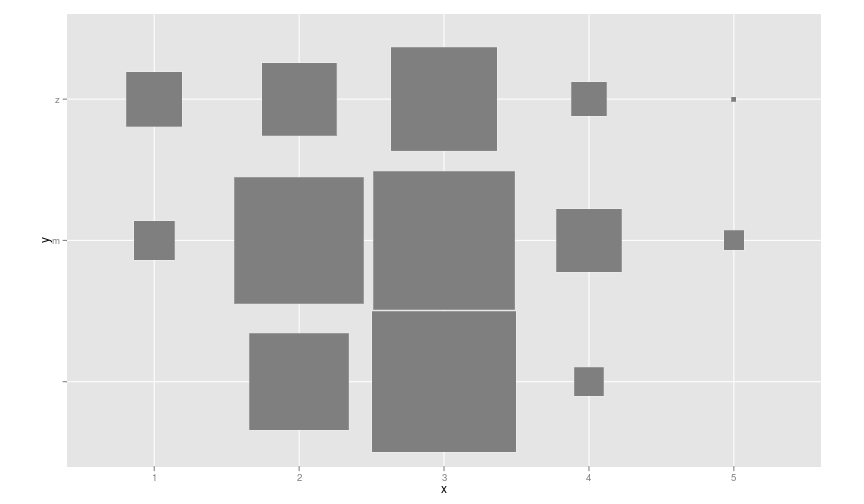``````(svyby(~dobrobitUhran, by = ~pol, design = svy, svymean, na.rm = T))  #
``````
``````##   pol dobrobitUhran      se
##               2.716 0.07107
## m   m         2.653 0.08381
## z   z         2.502 0.13801
``````
``````svyby(~dobrobitUhran, by = ~pol, design = svy, svymean, na.rm = T)
``````
``````##   pol dobrobitUhran      se
##               2.716 0.07107
## m   m         2.653 0.08381
## z   z         2.502 0.13801
``````
``````print(ftable(svyby(~dobrobitUhran, by = ~pol, design = svy, svymean,
na.rm = T)))
``````
``````##              dobrobitUhran
## pol
##     svymean        2.71574
##     SE             0.07107
## m   svymean        2.65349
##     SE             0.08381
## z   svymean        2.50235
##     SE             0.13801
``````
``````svyby(~counter, by = ~group, design = svy, svytotal, na.rm = T)  #very different total expected number of dogs per group
``````
``````##   group counter     se
## A     A  2013.6  977.0
## B     B  1665.2  776.1
## C     C   505.5  270.4
## D     D   458.6  254.0
## E     E   969.4  478.8
## F     F  2312.9  999.0
## G     G  2660.8 1025.8
## H     H   582.5  283.2
``````
``````
#
# ggfluctuation((svyby(~counter,by=~group,design=svy,svytotal,na.rm=T)[,-2]),'size')

``````

We can print out contingency tables as well.

``````print(xtable(svytable(~counter + group, svy)), "html")
``````
V1 A B C D E F G H
0 0.00 220.90 191.79 113.48 98.27 355.95 176.72 2.51 324.33
1 0.00 2013.61 1665.20 505.53 458.55 969.43 2312.91 2660.80 582.50
``````ggfluctuation((svytable(~counter + group, svy)), "size")
``````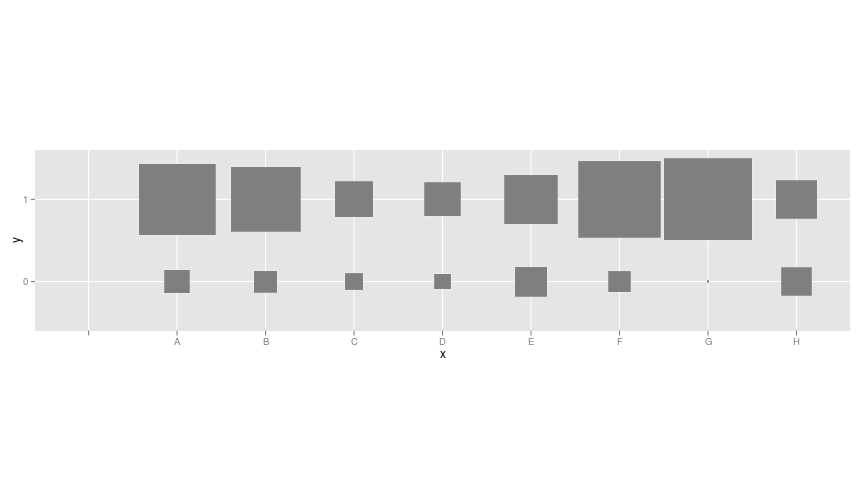``````print(xtable(svytable(~pol + starost, svy)), "html")
``````
V1 o s
1569.80 1709.10 2138.61
m 127.51 4109.54 532.39
z 88.36 1863.84 513.34
``````ggfluctuation((svytable(~pol + starost, svy)), "size")  #larger proportion of pups are female
``````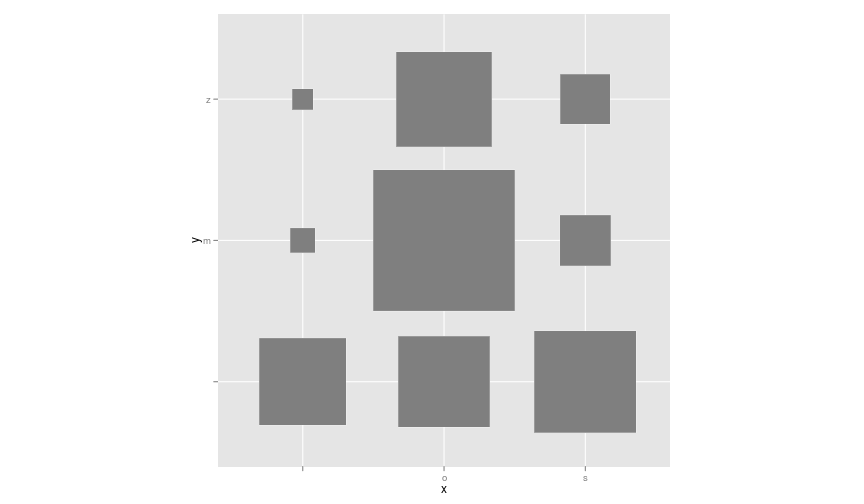``````print(xtable(svytable(~hour + starost, svy)), "html")
``````
V1 o s
4 282.66 1610.13 1311.77
5 498.53 1774.71 435.30
6 748.40 1832.61 372.99
7 246.02 2177.35 770.10
8 10.05 287.69 294.18
``````ggfluctuation((svytable(~hour + starost, svy)), "size")
``````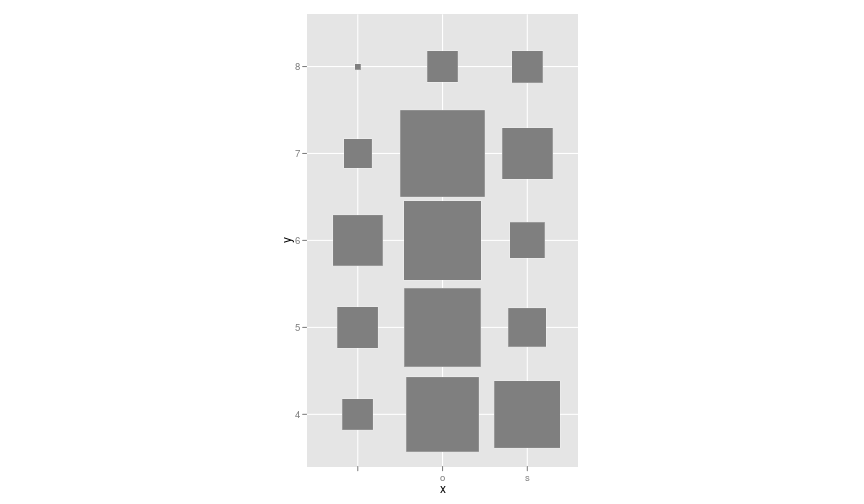``````print(xtable(svytable(~hour + day, svy)), "html")
``````
1 2 3
4 831.45 1959.38 413.74
5 1234.51 719.43 754.61
6 1428.27 919.81 605.92
7 928.81 1701.84 562.81
8 591.92 0.00 0.00
``````ggfluctuation((svytable(~hour + day, svy)), "size")
``````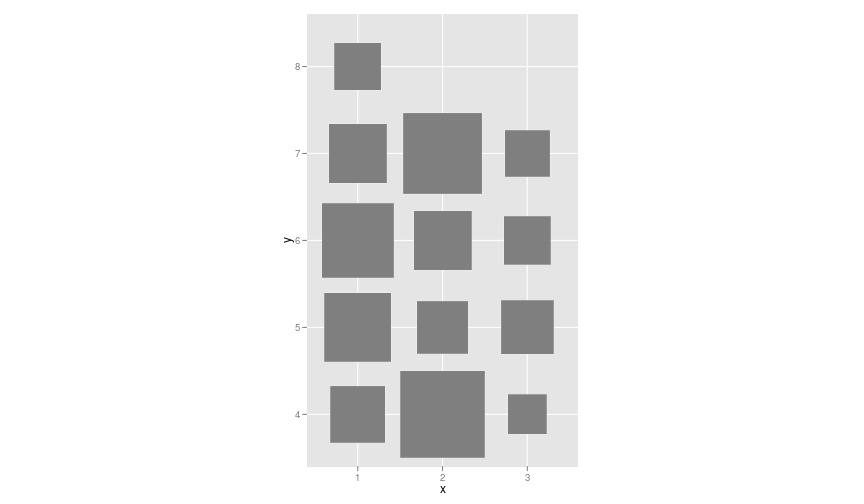``````print(xtable(svytable(~starost + group, svy)), "html")
``````
V1 A B C D E F G H
0.00 220.90 360.97 199.33 98.27 355.95 181.74 44.18 324.33
o 0.00 1118.51 1074.33 414.66 430.91 660.18 1333.56 2138.19 512.14
s 0.00 895.10 421.69 5.03 27.64 309.25 974.33 480.95 70.35
``````ggfluctuation((svytable(~starost + group, svy)), "size")  #groups c,d and h saw proportionatly more pups or classified more as puts
``````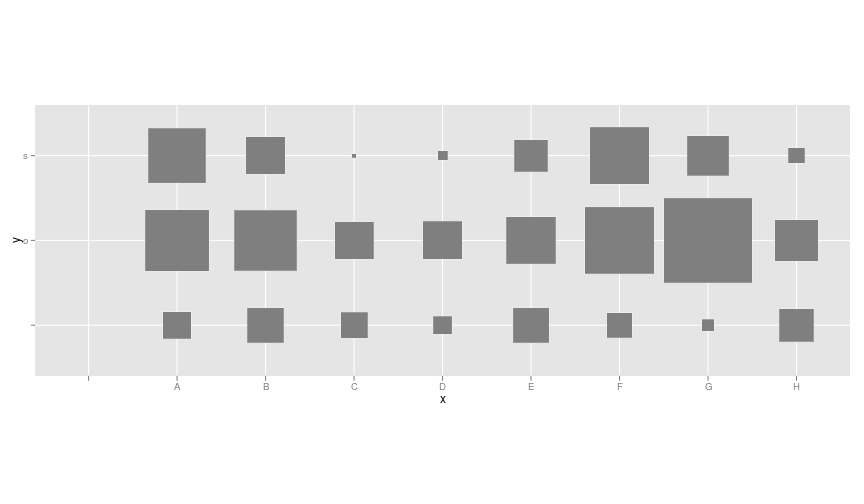``````print(xtable(svytable(~starost + dobrobitHramlje, svy)), "html")
``````
0 1
6.21 5.03
o 3505.97 639.80
s 1476.55 0.00
``````ggfluctuation((svytable(~starost + dobrobitHramlje, svy)), "size")  #only older dogs are limping
``````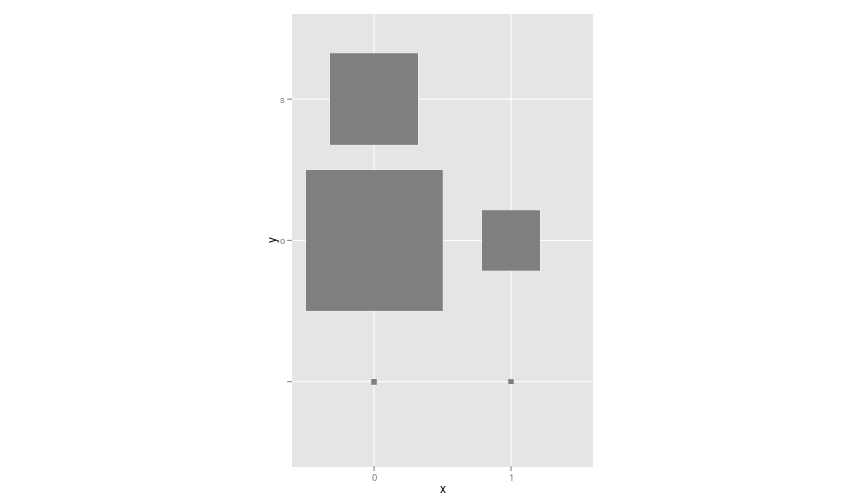``````print(xtable(svytable(~starost + dobrobitReakcija, svy)), "html")
``````
0 1 2 3 4 5
0.00 2.51 1.19 0.00 5.03 0.00
o 41.67 1749.67 1297.74 548.93 1825.88 196.82
s 0.00 809.76 243.51 61.77 848.41 250.00
``````ggfluctuation((svytable(~starost + dobrobitReakcija, svy)), "size")
``````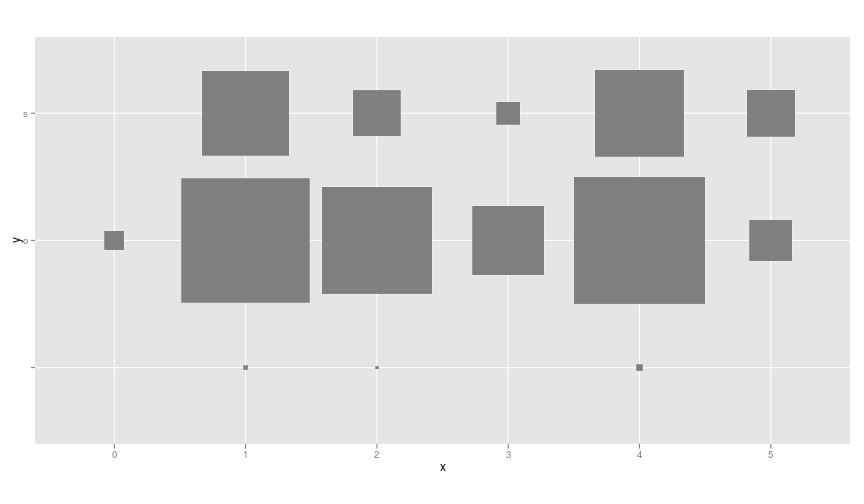``````print(xtable(svytable(~starost + reproduktivniStatusOK, svy)), "html")
``````
0 1
0.00 41.67
o 201.84 969.43
s 2.51 85.85
``````ggfluctuation((svytable(~starost + reproduktivniStatusOK, svy)),
"size")
``````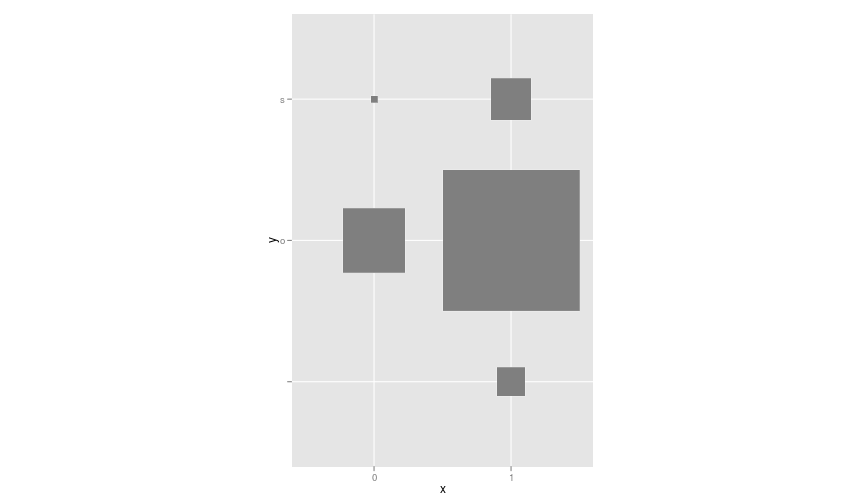``````print(xtable(svytable(~pol + reproduktivniStatusOK, svy)), "html")
``````
0 1
2.51 44.18
m 100.92 753.56
z 100.92 299.20
``````ggfluctuation((svytable(~pol + reproduktivniStatusOK, svy)), "size")
``````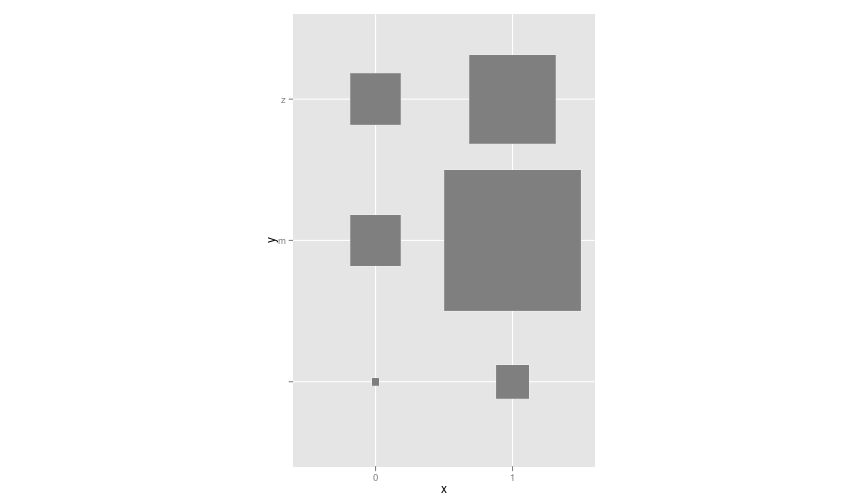``````# We could print overview maps, but we won't# load(file='mainmapL') mainmapBL <- openproj(mainmapB, projection =# '+proj=longlat') #convert to lat long. This is pretty important. The# other way would be to convert latlong coordinates to openstreetmap# format e.g.  projectMercator(xmin,ymin)# autoplot(mainmapL)+stat_contour(data=na.omit(combg),aes(y=lat,x=lon,z=x))# autoplot(mainmapL)+stat_bin2d(data=comb,aes(y=lat,x=lon))
``````

Here is the start of the data file

``````print(xtable(data.frame(data[1:4, ])), "html")
``````
clan level square dogNumber starost pol reproduktivniStatusLak reproduktivniStatusOK dobrobitHramlje dobrobitUhran Problemi.sa.kožom dobrobitReakcija označen opaznja X X.1 X.2 counter
1 MravDalila 1 48 1 o m 1 3 4 0 1.00
2 MravDalila 1 48 1 o m 1 3 4 0 1.00
3 MravDalila 1 48 1 o m 0 2 4 0 1.00
4 MravDalila 1 48 1 o m 0 3 4 0 1.00

Here is the start of the blocks-for-data file

``````print(xtable(data.frame(blocks[1:4, ])), "html")
``````
level square lat lon hour day group X
1 0 1 43.88 18.27 4 2 A
2 0 2 43.88 18.27 5 2 F
3 0 3 43.89 18.28 4 2 F
4 0 4 43.85 18.29 6 3 E

Here is how they are combined

``````print(xtable(data.frame(comb[1:4, 1:8])), "html")
``````
level square X clan dogNumber starost pol reproduktivniStatusLak
1 0 1 AH 1 s
2 0 1 AH 1 o z
3 0 1 ED 1 o z 1
4 0 1 AH 1 s
reproduktivniStatusOK dobrobitHramlje dobrobitUhran Problemi.sa.kožom dobrobitReakcija označen opaznja X.1 X.2 counter lat lon hour day group fpc
1 0 3 0 1 1.00 43.88 18.27 4 2 A 0.02
2 1 0 3 0 2 1 1.00 43.88 18.27 4 2 A 0.02
3 0 3 0 4 1 1.00 43.88 18.27 4 2 A 0.02
4 0 3 0 1 1.00 43.88 18.27 4 2 A 0.02